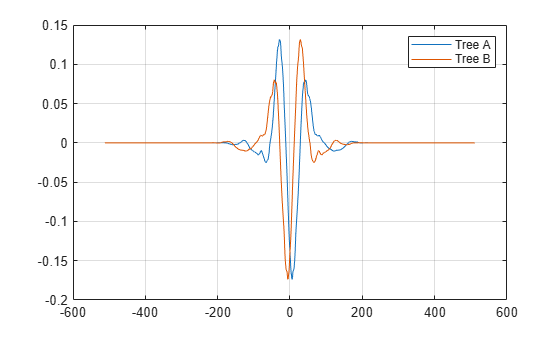qorthwavf

Kingsbury Q-shift filters

Description

example

[LoDa,LoDb,HiDa,HiDb,LoRa,LoRb,HiRa,HiRb] = qorthwavf(num) returns the Kingsbury Q-shift filters for the Q-shift complex dual-tree transform. The integer num refers to the number of nonzero coefficients (taps) in the filter. Valid options for num are 6, 10, 14, 16, and 18. All filters are of even lengths and the tree B filters are the time reverse of the tree A filters.

Examples

collapse all

Obtain the Q-shift filters for the case with 10 nonzero coefficients.

[LoDa,LoDb,HiDa,HiDb,LoRa,LoRb,HiRa,HiRb] = qorthwavf(10);

Use the dwtfilterbank function and create two discrete wavelet transform filter banks. Use the tree A analysis filters in the first filter bank, and the tree B analysis filters in the second filter bank.

fbTreeA = dwtfilterbank('Wavelet','Custom',...
'CustomScalingFilter',LoDa,...
'CustomWaveletFilter',HiDa);
fbTreeB = dwtfilterbank('Wavelet','Custom',...
'CustomScalingFilter',LoDb,...
'CustomWaveletFilter',HiDb);

Plot the coarsest-scale wavelets of each filter bank.

[psiA,t] = wavelets(fbTreeA);
[psiB,~] = wavelets(fbTreeB);
plot(t,psiA(end,:))
hold on
plot(t,psiB(end,:))
grid on
hold off
legend('Tree A','Tree B')Confirm both filter banks are orthogonal.

isOrthogonal(fbTreeA)
ans = logical
1

isOrthogonal(fbTreeB)
ans = logical
1

Input Arguments

collapse all

Number of nonzero coefficients in the Kingsbury Q-shift filters, specified as one of the listed values.

Output Arguments

collapse all

Tree A lowpass (scaling) analysis filter associated with the Q-shift filter, returned as a real-valued vector.

Tree B lowpass (scaling) analysis filter associated with the Q-shift filter, returned as a real-valued vector.

Tree A highpass (wavelet) analysis filter associated with the Q-shift filter, returned as a real-valued vector.

Tree B highpass (wavelet) analysis filter associated with the Q-shift filter, returned as a real-valued vector.

Tree A lowpass (scaling) synthesis filter associated with the Q-shift filter, returned as a real-valued vector.

Tree B lowpass (scaling) synthesis filter associated with the Q-shift filter, returned as a real-valued vector.

Tree A highpass (wavelet) synthesis filter associated with the Q-shift filter, returned as a real-valued vector.

Tree B highpass (wavelet) synthesis filter associated with the Q-shift filter, returned as a real-valued vector.

 Antonini, M., M. Barlaud, P. Mathieu, and I. Daubechies. “Image Coding Using Wavelet Transform.” IEEE Transactions on Image Processing 1, no. 2 (April 1992): 205–20. https://doi.org/10.1109/83.136597.

 Kingsbury, Nick. “Complex Wavelets for Shift Invariant Analysis and Filtering of Signals.” Applied and Computational Harmonic Analysis 10, no. 3 (May 2001): 234–53. https://doi.org/10.1006/acha.2000.0343.

 Le Gall, D., and A. Tabatabai. “Sub-Band Coding of Digital Images Using Symmetric Short Kernel Filters and Arithmetic Coding Techniques.” In ICASSP-88., International Conference on Acoustics, Speech, and Signal Processing, 761–64. New York, NY, USA: IEEE, 1988. https://doi.org/10.1109/ICASSP.1988.196696.

Wavelet Toolbox DocumentationGet trial now